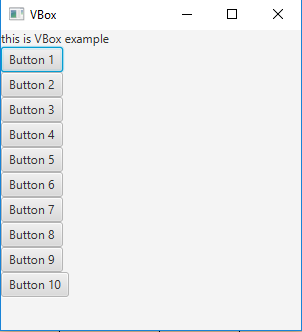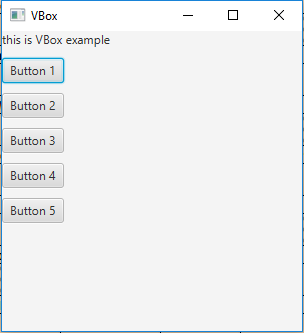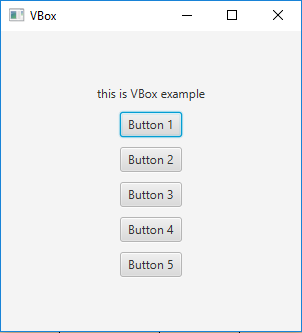# JavaFX | VBox Class

VBox is a part of JavaFX. VBox lays out its children in form of vertical columns. If the vbox has a border and/or padding set, then the contents will be layed out within those insets. VBox class extends Pane class.

Constructor of the class:

1. VBox(): Creates a VBox layout with spacing = 0 and alignment at TOP_LEFT.
2. VBox(double s): Creates a new VBox with specified spacing between children.
3. VBox(double s, Node… c): Creates a new VBox with specified nodes and spacing between them.
4. VBox(Node… c): Creates an VBox layout with spacing = 0.

Commonly Used Methods:

Method Explanation
getAlignment() Returns the value of property alignment.
getSpacing() Returns the spacing between its children.
setAlignment(Pos value) Sets the Alignment of the VBox.
getChildren() Returns the nodes in the VBox.

Below programs illustrate the use of VBox class:

1. Java Program to create a VBox and add it to the stage: In this program we will create a VBox named vbox. We will create a label and add it to the vbox. We will also create some buttons and add them to the VBox using the getChildren().add() function. Now create a scene and add the vbox to the scene and add the scene to the stage and call show() function to display the final results.

 `// Java Program to create a VBox  ` `// and add it to the stage ` `import` `javafx.application.Application; ` `import` `javafx.scene.Scene; ` `import` `javafx.scene.control.*; ` `import` `javafx.scene.layout.*; ` `import` `javafx.stage.Stage; ` `import` `javafx.event.ActionEvent; ` `import` `javafx.event.EventHandler; ` `import` `javafx.scene.canvas.*; ` `import` `javafx.scene.web.*; ` `import` `javafx.scene.Group; ` ` `  `public` `class` `VBOX_1 ``extends` `Application { ` ` `  `    ``// launch the application ` `    ``public` `void` `start(Stage stage) ` `    ``{ ` ` `  `        ``try` `{ ` ` `  `            ``// set title for the stage ` `            ``stage.setTitle(``"VBox"``); ` ` `  `            ``// create a VBox ` `            ``VBox vbox = ``new` `VBox(); ` ` `  `            ``// create a label ` `            ``Label label = ``new` `Label(``"this is VBox example"``); ` ` `  `            ``// add label to vbox ` `            ``vbox.getChildren().add(label); ` ` `  `            ``// add buttons to VBox ` `            ``for` `(``int` `i = ``0``; i < ``10``; i++) ` `            ``{ ` `                ``vbox.getChildren().add(``new` `Button(``"Button "` `+ (``int``)(i + ``1``))); ` `            ``} ` ` `  `            ``// create a scene ` `            ``Scene scene = ``new` `Scene(vbox, ``300``, ``300``); ` ` `  `            ``// set the scene ` `            ``stage.setScene(scene); ` ` `  `            ``stage.show(); ` `        ``} ` ` `  `        ``catch` `(Exception e) { ` ` `  `            ``System.out.println(e.getMessage()); ` `        ``} ` `    ``} ` ` `  `    ``// Main Method ` `    ``public` `static` `void` `main(String args[]) ` `    ``{ ` ` `  `        ``// launch the application ` `        ``launch(args); ` `    ``} ` `} `

Output:2. Java Program to create a VBox, add spaces between its elements and add it to the stage: In this program we will create a VBox named vbox. We will set the spacing by passing a double value of space as an argument to the constructor. Now create a label and add it to the vbox. To add some buttons to the VBox use the getChildren().add() function. Finally, create a scene and add the vbox to the scene and add the scene to the stage and call show() function to display the final results.

 `// Java Program to create a VBox, add  ` `// spaces between its elements and add ` `// it to the stage ` `import` `javafx.application.Application; ` `import` `javafx.scene.Scene; ` `import` `javafx.scene.control.*; ` `import` `javafx.scene.layout.*; ` `import` `javafx.stage.Stage; ` `import` `javafx.event.ActionEvent; ` `import` `javafx.event.EventHandler; ` `import` `javafx.scene.canvas.*; ` `import` `javafx.scene.web.*; ` `import` `javafx.scene.Group; ` ` `  `public` `class` `VBOX_2 ``extends` `Application { ` ` `  `    ``// launch the application ` `    ``public` `void` `start(Stage stage) ` `    ``{ ` ` `  `        ``try` `{ ` ` `  `            ``// set title for the stage ` `            ``stage.setTitle(``"VBox"``); ` ` `  `            ``// create a VBox ` `            ``VBox vbox = ``new` `VBox(``10``); ` ` `  `            ``// create a label ` `            ``Label label = ``new` `Label(``"this is VBox example"``); ` ` `  `            ``// add label to vbox ` `            ``vbox.getChildren().add(label); ` ` `  `            ``// add buttons to VBox ` `            ``for` `(``int` `i = ``0``; i < ``5``; i++) ` `            ``{ ` `                ``vbox.getChildren().add(``new` `Button(``"Button "` `+ (``int``)(i + ``1``))); ` `            ``} ` ` `  `            ``// create a scene ` `            ``Scene scene = ``new` `Scene(vbox, ``300``, ``300``); ` ` `  `            ``// set the scene ` `            ``stage.setScene(scene); ` ` `  `            ``stage.show(); ` `        ``} ` ` `  `        ``catch` `(Exception e) { ` ` `  `            ``System.out.println(e.getMessage()); ` `        ``} ` `    ``} ` ` `  `    ``// Main Method ` `    ``public` `static` `void` `main(String args[]) ` `    ``{ ` ` `  `        ``// launch the application ` `        ``launch(args); ` `    ``} ` `} `

Output:3. Java Program to create a VBox, add spaces between its elements, set an alignment and add it to the stage: In this program we will create a VBox named vbox. We will set the spacing by passing a double value of space as an argument to the constructor. Set the alignment of the VBox using the setAlignment() function. Then create a label and add it to the vbox. Add some buttons to the VBox using the getChildren().add() function. Finally, create a scene and add the vbox to the scene and add the scene to the stage and call show() function to display the final results.

 `// Java Program to create a VBox, add spaces ` `// between its elements, set an alignment  ` `// and add it to the stage ` `import` `javafx.application.Application; ` `import` `javafx.scene.Scene; ` `import` `javafx.scene.control.*; ` `import` `javafx.scene.layout.*; ` `import` `javafx.stage.Stage; ` `import` `javafx.event.ActionEvent; ` `import` `javafx.event.EventHandler; ` `import` `javafx.scene.Group; ` `import` `javafx.geometry.Pos; ` ` `  `public` `class` `VBOX_3 ``extends` `Application { ` ` `  `    ``// launch the application ` `    ``public` `void` `start(Stage stage) ` `    ``{ ` ` `  `        ``try` `{ ` ` `  `            ``// set title for the stage ` `            ``stage.setTitle(``"VBox"``); ` ` `  `            ``// create a VBox ` `            ``VBox vbox = ``new` `VBox(``10``); ` ` `  `            ``// create a label ` `            ``Label label = ``new` `Label(``"this is VBox example"``); ` ` `  `            ``// add label to vbox ` `            ``vbox.getChildren().add(label); ` ` `  `            ``// set alignment ` `            ``vbox.setAlignment(Pos.CENTER); ` ` `  `            ``// add buttons to VBox ` `            ``for` `(``int` `i = ``0``; i < ``5``; i++) ` `            ``{ ` `                ``vbox.getChildren().add(``new` `Button(``"Button "` `+ (``int``)(i + ``1``))); ` `            ``} ` ` `  `            ``// create a scene ` `            ``Scene scene = ``new` `Scene(vbox, ``300``, ``300``); ` ` `  `            ``// set the scene ` `            ``stage.setScene(scene); ` ` `  `            ``stage.show(); ` `        ``} ` ` `  `        ``catch` `(Exception e) { ` ` `  `            ``System.out.println(e.getMessage()); ` `        ``} ` `    ``} ` ` `  `    ``// Main Method ` `    ``public` `static` `void` `main(String args[]) ` `    ``{ ` ` `  `        ``// launch the application ` `        ``launch(args); ` `    ``} ` `} `

Output:Note: The above programs might not run in an online IDE please use an offline compiler.

Attention reader! Don’t stop learning now. Get hold of all the important Java and Collections concepts with the Fundamentals of Java and Java Collections Course at a student-friendly price and become industry ready.

My Personal Notes arrow_drop_upThird year Department of Information Technology Jadavpur University

If you like GeeksforGeeks and would like to contribute, you can also write an article using contribute.geeksforgeeks.org or mail your article to contribute@geeksforgeeks.org. See your article appearing on the GeeksforGeeks main page and help other Geeks.

Please Improve this article if you find anything incorrect by clicking on the "Improve Article" button below.

Article Tags :
Practice Tags :

Be the First to upvote.

Please write to us at contribute@geeksforgeeks.org to report any issue with the above content.